# The HPFMM Procedure

### PROC HPFMM Statement

• PROC HPFMM <options>;

The PROC HPFMM statement invokes the HPFMM procedure. Table 6.2 summarizes the options available in the PROC HPFMM statement. These and other options in the PROC HPFMM statement are then described fully in alphabetical order.

Table 6.2: PROC HPFMM Statement Options

Option

Description

Basic Options

Specifies the input data set

Specifies how the procedure responds to support violations in the data

Specifies the length of effect names

Specifies the random number seed for analyses that require random number draws

Displayed Output

Displays information about the mixture components

Displays the asymptotic correlation matrix of the maximum likelihood parameter estimates or the empirical correlation matrix of the Bayesian posterior estimates

Displays the asymptotic covariance matrix of the maximum likelihood parameter estimates or the empirical covariance matrix of the Bayesian posterior estimates

Displays the inverse of the covariance matrix of the parameter estimates

Displays fit information for all examined models

Suppresses the "Class Level Information" table completely or partially

Suppresses the "Iteration History Information" table

Suppresses tabular and graphical output

Specifies how parameters are displayed in ODS tables

Produces ODS statistical graphics

Computational Options

Specifies the criterion used in model selection

Prevents centering and scaling of the regressor variables

Specifies a variable that defines a partial classification

Options Related to Optimization

Tunes an absolute function convergence criterion

Tunes an absolute function difference convergence criterion

Tunes the absolute gradient convergence criterion

Specifies a relative function convergence criterion that is based on a relative change of the function value

Specifies a relative function convergence criterion that is based on a predicted reduction of the objective function

Tunes the relative gradient convergence criterion

Specifies the maximum number of iterations in any optimization

Specifies the maximum number of function evaluations in any optimization

Specifies the upper limit of CPU time in seconds for any optimization

Specifies the minimum number of iterations in any optimization

Selects the optimization technique

Singularity Tolerances

Tunes the value assigned to an invalid component log likelihood

Tunes singularity for Cholesky decompositions

Tunes singularity for the residual variance

Tunes general singularity criterion

You can specify the following options in the PROC HPFMM statement.

ABSCONV=r
ABSTOL=r

specifies an absolute function convergence criterion. For minimization, the termination criterion is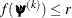, where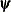is the vector of parameters in the optimization and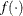is the objective function. The default value of r is the negative square root of the largest double-precision value, which serves only as a protection against overflows.

ABSFCONV=r <n>
ABSFTOL=r<n>

specifies an absolute function difference convergence criterion. For all techniques except NMSIMP, the termination criterion is a small change of the function value in successive iterations: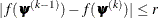Here,denotes the vector of parameters that participate in the optimization, andis the objective function. The same formula is used for the NMSIMP technique, but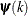is defined as the vertex with the lowest function value, and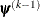is defined as the vertex with the highest function value in the simplex. The default value is r=0. The optional integer value n specifies the number of successive iterations for which the criterion must be satisfied before the process can be terminated.

ABSGCONV=r <n>
ABSGTOL=r<n>

specifies an absolute gradient convergence criterion. The termination criterion is a small maximum absolute gradient element: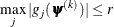Here,denotes the vector of parameters that participate in the optimization, and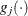is the gradient of the objective function with respect to the jth parameter. This criterion is not used by the NMSIMP technique. The default value is r=1E–5. The optional integer value n specifies the number of successive iterations for which the criterion must be satisfied before the process can be terminated.

COMPONENTINFO
COMPINFO
CINFO

COV

produces the covariance matrix of the parameter estimates. For maximum likelihood estimation, this matrix is based on the inverse (projected) Hessian matrix. For Bayesian estimation, it is the empirical covariance matrix of the posterior estimates. The covariance matrix is shown for all parameters, even if they did not participate in the optimization or sampling.

COVI

produces the inverse of the covariance matrix of the parameter estimates. For maximum likelihood estimation, the covariance matrix is based on the inverse (projected) Hessian matrix. For Bayesian estimation, it is the empirical covariance matrix of the posterior estimates. This matrix is then inverted by sweeping, and rows and columns that correspond to linear dependencies or singularities are zeroed.

CORR

produces the correlation matrix of the parameter estimates. For maximum likelihood estimation this matrix is based on the inverse (projected) Hessian matrix. For Bayesian estimation, it is based on the empirical covariance matrix of the posterior estimates.

CRITERION=keyword
CRIT=keyword

specifies the criterion by which the HPFMM procedure ranks models when multiple models are evaluated during maximum likelihood estimation. You can choose from the following keywords to rank models:

AIC

based on Akaike’s information criterion

AICC

based on the bias-corrected AIC criterion

BIC

based on the Bayesian information criterion

based on the largest element of the gradient (in absolute value)

LOGL | LL

based on the mixture log likelihood

PEARSON

based on the Pearson statistic

The default is CRITERION=BIC.

DATA=SAS-data-set

names the SAS data set to be used by PROC HPFMM. The default is the most recently created data set.

EXCLUSION=NONE | ANY | ALL
EXCLUDE=NONE | ANY | ALL

specifies how the HPFMM procedure handles support violations of observations. For example, in a mixture of two Poisson variables, negative response values are not possible. However, in a mixture of a Poisson and a normal variable, negative values are possible, and their likelihood contribution to the Poisson component is zero. An observation that violates the support of one component distribution of the model might be a valid response with respect to one or more other component distributions. This requires some nuanced handling of support violations in mixture models.

The default exclusion technique, EXCLUSION=ALL, removes an observation from the analysis only if it violates the support of all component distributions. The other extreme, EXCLUSION=NONE, permits an observation into the analysis regardless of support violations. EXCLUSION=ANY removes observations from the analysis if the response violates the support of any component distributions. In the single-component case, EXCLUSION=ALL and EXCLUSION=ANY are identical.

FCONV=r<n>
FTOL=r<n>

specifies a relative function convergence criterion that is based on the relative change of the function value. For all techniques except NMSIMP, PROC HPFMM terminates when there is a small relative change of the function value in successive iterations: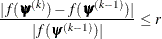Here,denotes the vector of parameters that participate in the optimization, andis the objective function. The same formula is used for the NMSIMP technique, but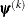is defined as the vertex with the lowest function value, andis defined as the vertex with the highest function value in the simplex.

The default is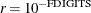, where FDIGITS is by default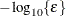, andis the machine precision. The optional integer value n specifies the number of successive iterations for which the criterion must be satisfied before the process terminates.

FCONV2=r<n>
FTOL2=r<n>

specifies a relative function convergence criterion that is based on the predicted reduction of the objective function. For all techniques except NMSIMP, the termination criterion is a small predicted reduction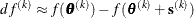of the objective function. The predicted reduction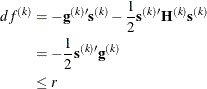is computed by approximating the objective function f by the first two terms of the Taylor series and substituting the Newton step: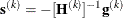For the NMSIMP technique, the termination criterion is a small standard deviation of the function values of the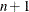simplex vertices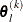,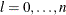,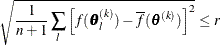where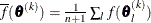. If there are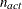boundary constraints active at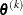, the mean and standard deviation are computed only for the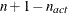unconstrained vertices.

The default value is r = 1E–6 for the NMSIMP technique and r = 0 otherwise. The optional integer value n specifies the number of successive iterations for which the criterion must be satisfied before the process terminates.

FITDETAILS

requests that the "Optimization Information," "Iteration History," and "Fit Statistics" tables be produced for all optimizations when models with different number of components are evaluated. For example, the following statements fit a binomial regression model with up to three components and produces fit and optimization information for all three:

proc hpfmm fitdetails;
model y/n  = x / kmax=3;
run;


Without the FITDETAILS option, only the "Fit Statistics" table for the selected model is displayed.

In Bayesian estimation, the FITDETAILS option displays the following tables for each model that the procedure fits: "Bayes Information," "Iteration History," "Prior Information," "Fit Statistics," "Posterior Summaries," "Posterior Intervals," and any requested diagnostics tables. The "Iteration History" table appears only if the BAYES statement includes the INITIAL= MLE option.

Without the FITDETAILS option, these tables are listed only for the selected model.

GCONV=r<n>
GTOL=r<n>

specifies a relative gradient convergence criterion. For all techniques except CONGRA and NMSIMP, the termination criterion is a small normalized predicted function reduction: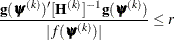Here,denotes the vector of parameters that participate in the optimization,is the objective function, and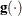is the gradient. For the CONGRA technique (where a reliable Hessian estimateis not available), the following criterion is used: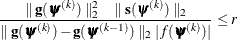This criterion is not used by the NMSIMP technique. The default value is r=1E–8. The optional integer value n specifies the number of successive iterations for which the criterion must be satisfied before the process can terminate.

HESSIAN

displays the Hessian matrix of the model. This option is not available for Bayesian estimation.

INVALIDLOGL=r

specifies the value assumed by the HPFMM procedure if a log likelihood cannot be computed (for example, because the value of the response variable falls outside of the response distribution’s support). The default value is –1E20.

ITDETAILS

adds parameter estimates and gradients to the "Iteration History" table. If the HPFMM procedure centers or scales the model variables (or both), the parameter estimates and gradients reported during the iteration refer to that scale. You can suppress centering and scaling with the NOCENTER option.

MAXFUNC=n
MAXFU=n

specifies the maximum number of function calls in the optimization process. The default values are as follows, depending on the optimization technique:

• TRUREG, NRRIDG, and NEWRAP: 125

• QUANEW and DBLDOG: 500

• CONGRA: 1000

• NMSIMP: 3000

The optimization can terminate only after completing a full iteration. Therefore, the number of function calls that are actually performed can exceed the number that is specified by the MAXFUNC= option. You can choose the optimization technique with the TECHNIQUE= option.

MAXITER=n
MAXIT=n

specifies the maximum number of iterations in the optimization process. The default values are as follows, depending on the optimization technique:

• TRUREG, NRRIDG, and NEWRAP: 50

• QUANEW and DBLDOG: 200

• CONGRA: 400

• NMSIMP: 1000

These default values also apply when n is specified as a missing value. You can choose the optimization technique with the TECHNIQUE= option.

MAXTIME=r

specifies an upper limit of r seconds of CPU time for the optimization process. The time is checked only at the end of each iteration. Therefore, the actual run time might be longer than the specified time. By default, CPU time is not limited.

MINITER=n
MINIT=n

specifies the minimum number of iterations. The default value is 0. If you request more iterations than are actually needed for convergence to a stationary point, the optimization algorithms can behave strangely. For example, the effect of rounding errors can prevent the algorithm from continuing for the required number of iterations.

NAMELEN=number

specifies the length to which long effect names are shortened. The default and minimum value is 20.

NOCENTER

requests that regressor variables not be centered or scaled. By default the HPFMM procedure centers and scales columns of thematrix if the models contain intercepts. If NOINT options in MODEL statements are in effect, the columns ofare scaled but not centered. Centering and scaling can help with the stability of estimation and sampling algorithms. The HPFMM procedure does not produce a table of the centered and scaled coefficients and provides no user control over the type of centering and scaling that is applied. The NOCENTER option turns any centering and scaling off and processes the raw values of the continuous variables.

NOCLPRINT<=number>

suppresses the display of the "Class Level Information" table if you do not specify number. If you specify number, the values of the classification variables are displayed for only those variables whose number of levels is less than number. Specifying a number helps to reduce the size of the "Class Level Information" table if some classification variables have a large number of levels.

NOITPRINT

suppresses the display of the "Iteration History Information" table.

NOPRINT

suppresses the normal display of tabular and graphical results. The NOPRINT option is useful when you want to create only one or more output data sets with the procedure. This option temporarily disables the Output Delivery System (ODS); see Chapter 20: Using the Output Delivery System in SAS/STAT 14.1 User's Guide, for more information.

PARMSTYLE=EFFECT | LABEL

specifies the display style for parameters and effects. The HPFMM procedure can display parameters in two styles:

• The EFFECT style (which is used by the MIXED and GLIMMIX procedure, for example) identifies a parameter with an "Effect" column and adds separate columns for the CLASS variables in the model.

• The LABEL style creates one column, named Parameter, that combines the relevant information about a parameter into a single column. If your model contains multiple CLASS variables, the LABEL style might use space more economically.

The EFFECT style is the default for models that contain effects; otherwise the LABEL style is used (for example, in homogeneous mixtures). You can change the display style with the PARMSTYLE= option. Regardless of the display style, ODS output data sets that contain information about parameter estimates contain columns for both styles.

PARTIAL=variable
MEMBERSHIP=variable

specifies a variable in the input data set that identifies component membership. You can specify missing values for observations whose component membership is undetermined; this is known as a partial classification (McLachlan and Peel 2000, p. 75). For observations with known membership, the likelihood contribution is no longer a mixture. If observation i is known to be a member of component m, then its log likelihood contribution is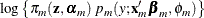Otherwise, if membership is undetermined, it is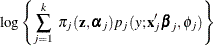The variable specified in the PARTIAL= option can be numeric or character. In case of a character variable, the variable must appear in the CLASS statement. If the PARTIAL= variable appears in the CLASS statement, the membership assignment is made based on the levelized values of the variable, as shown in the "Class Level Information" table. Invalid values of the PARTIAL= variable are ignored.

In a model in which label switching is a problem, the switching can sometimes be avoided by assigning just a few observations to categories. For example, in a three-component model, switches might be prevented by assigning the observation with the smallest response value to the first component and the observation with the largest response value to the last component.

PLOTS <(global-plot-options)> <=plot-request <(options)>>
PLOTS <(global-plot-options)> <=(plot-request <(options)> <... plot-request <(options)>>)>

controls the plots produced through ODS Graphics.

ODS Graphics must be enabled before plots can be requested. For example:

ods graphics on;
proc hpfmm data=yeast seed=12345;
model count/n = / k=2;
freq f;
bayes;
run;
ods graphics off;


Global Plot Options

The global-plot-options apply to all relevant plots generated by the HPFMM procedure. The global-plot-options supported by the HPFMM procedure are as follows:

UNPACKPANEL
UNPACK

displays each graph separately. (By default, some graphs can appear together in a single panel.)

ONLY

produces only the specified plots. This option is useful if you do not want the procedure to generate all default graphics, but only the ones specified.

Specific Plot Options

The following listing describes the specific plots and their options.

ALL

requests that all plots appropriate for the analysis be produced.

NONE

requests that no ODS graphics be produced.

DENSITY <(density-options)>

requests a plot of the data histogram and mixture density function. This graphic is a default graphic in models without effects in the MODEL statements and is available only in these models. Furthermore, all distributions involved in the mixture must be continuous. You can specify the following density-options to modify the plot:

CUMULATIVE
CDF

displays the histogram and densities in cumulative form.

NBINS=n
BINS=n

specifies the number of bins in the histogram; n is greater than or equal to 0. By default, the HPFMM procedure computes a suitable bin width and number of bins, based on the range of the response and the number of usable observations. The option has no effect for binary data.

NOCOMPONENTS
NOCOMP

suppresses the component densities from the plot. If the component densities are displayed, they are scaled so that their sum equals the mixture density at any point on the graph. In single-component models, this option has no effect.

NODENSITY
NODENS

suppresses the computation of the mixture density (and the component densities if the COMPONENTS suboption is specified). If you specify the NOHISTOGRAM and the NODENSITY option, no graphic is produced.

NOLABEL

suppresses the component identification with labels. By default, the HPFMM procedure labels component densities in the legend of the plot. If you do not specify a model label with the LABEL= option in the MODEL statement, an identifying label is constructed from the parameter estimates that are associated with the component. In this case the parameter values are not necessarily the mean and variance of the distribution; the values used to identify the densities on the plot are chosen to simplify linking between graphical and tabular results.

NOHISTOGRAM
NOHIST

suppresses the computation of the histogram of the raw values. If you specify the NOHISTOGRAM and the NODENSITY option, no graphic is produced.

NPOINTS=n
N=n

specifies the number of values used to compute the density functions; n is greater than or equal to 0. The default is N=200.

WIDTH=value
BINWIDTH=value

specifies the bin width for the histogram. The value is specified in units of the response variable and must be positive. The option has no effect for binary data.

requests a trace panel with posterior diagnostics for a Bayesian analysis. If a BAYES statement is present, the trace panel plots are generated by default, one for each sampled parameter. You can specify the following tadpanel-options to modify the graphic:

BOX
BOXPLOT

replaces the autocorrelation plot with a box plot of the posterior sample.

SMOOTH=NONE | MEAN | SPLINE

adds a reference estimate to the trace plot. By default, SMOOTH=NONE. SMOOTH=MEAN uses the arithmetic mean of the trace as the reference. SMOOTH=SPLINE adds a penalized B-spline.

REFERENCE= reference-style

adds vertical reference lines to the density plot, trace plot, and box plot. The available options for the reference-style are:

NONE

suppresses the reference lines

EQT

requests equal-tail intervals

HPD

requests intervals of highest posterior density. The level for the credible or HPD intervals is chosen based on the "Posterior Interval Statistics" table.

PERCENTILES

(or PERC) for percentiles. Up to three percentiles can be displayed, as based on the "Posterior Summary Statistics" table.

The default is REFERENCE=EQT.

UNPACK

unpacks the panel graphic and displays its elements as separate plots.

CRITERIONPANEL <(critpanel-options)>

requests a plot for comparing the model fit criteria for different numbers of components. This plot is available only if you also specify the KMAX option in at least one MODEL statement. The plot includes different criteria, depending on whether you are using maximum likelihood or Bayesian estimation. You can specify the following critpanel-option to modify the plot:

UNPACK

unpacks the panel plot and displays its elements as separate plots, one for each fit criterion.

SEED=n

determines the random number seed for analyses that depend on a random number stream. If you do not specify a seed or if you specify a value less than or equal to zero, the seed is generated from reading the time of day from the computer clock. The largest possible value for the seed is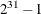. The seed value is reported in the "Model Information" table.

You can use the SYSRANDOM and SYSRANEND macro variables after a PROC HPFMM run to query the initial and final seed values. However, using the final seed value as the starting seed for a subsequent analysis does not continue the random number stream where the previous analysis left off. The SYSRANEND macro variable provides a mechanism to pass on seed values to ensure that the sequence of random numbers is the same every time you run an entire program.

Analyses that use the same (nonzero) seed are not completely reproducible if they are executed with a different number of threads since the random number streams in separate threads are independent. You can control the number of threads used by the HPFMM procedure with system options or through the PERFORMANCE statement in the HPFMM procedure.

SINGCHOL=number

tunes the singularity criterion in Cholesky decompositions. The default is 1E4 times the machine epsilon; this product is approximately 1E–12 on most computers.

SINGRES=number

sets the tolerance for which the residual variance or scale parameter is considered to be zero. The default is 1E4 times the machine epsilon; this product is approximately 1E–12 on most computers.

SINGULAR=number

tunes the general singularity criterion applied by the HPFMM procedure in sweeps and inversions. The default is 1E4 times the machine epsilon; this product is approximately 1E–12 on most computers.

TECHNIQUE=keyword
TECH=keyword

specifies the optimization technique to obtain maximum likelihood estimates. You can choose from the following techniques by specifying the appropriate keyword:

CONGRA

DBLDOG

performs a version of double-dogleg optimization.

NEWRAP

performs a Newton-Raphson optimization combining a line-search algorithm with ridging.

NMSIMP

NONE

performs no optimization.

NRRIDG

performs a Newton-Raphson optimization with ridging.

QUANEW

performs a dual quasi-Newton optimization.

TRUREG

performs a trust-region optimization.

The default is TECH=QUANEW.

For more details about these optimization methods, see the section Choosing an Optimization Algorithm.

ZEROPROB=number

tunes the threshold (a value between 0 and 1) below which the HPFMM procedure considers a component mixing probability to be zero. This affects the calculation of the number of effective components. The default is the square root of the machine epsilon; this is approximately 1E–8 on most computers.